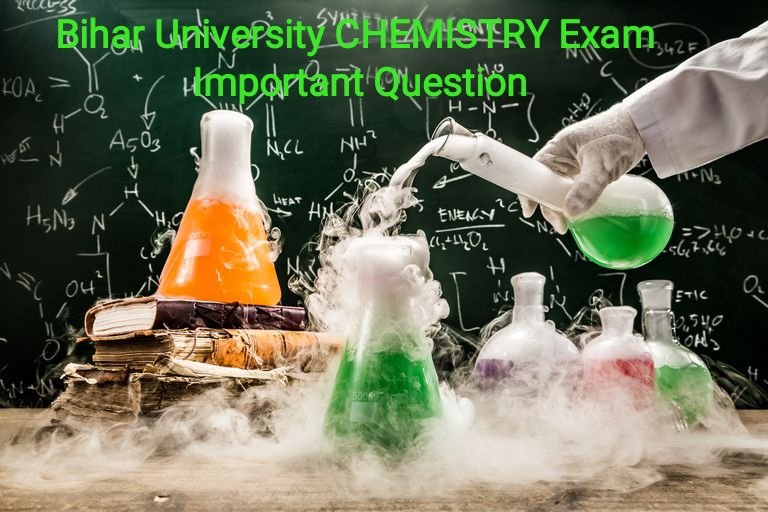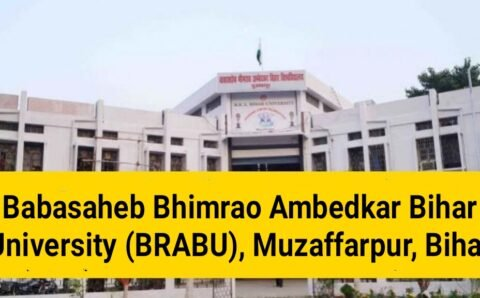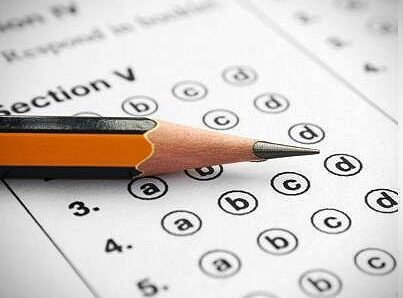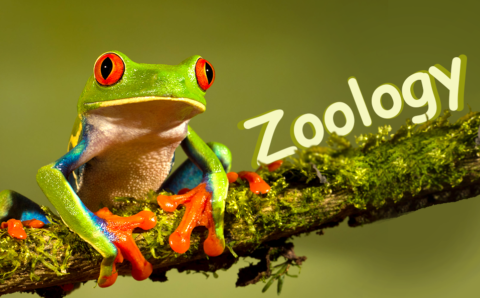# बिहार यूनिवर्सिटी (Muzaffarpur) CHEMISTRY Exam ImportantQuestionChemistry Exam Important Questions
(DAY 4) 11-03-2021

Graduation TDC Part 1 (2019-2022) Math/Physics/Chemistry

PG 1st semester (2019-21) – Physics / Math – Important questions

## Are you searching for BSc 1st year and PG 1st semester important questions in Physics/Math/Chemistry? Well, you are in the right place. In this post, we are go to share some of the most important questions of Physics/Math/Chemistry for TDC Part 1and PG 1st semester students. (B.R.A BIHAR UNIVERSITY)

TDC PART 1 CHEMISTRY (Hons.)
(Day 4)

(08-03-2021) TDC PART 1 CHEMISTRY (Hons.)

CHEMISTRY (Hons.) Paper-1

TDC Part 1 (2019-22)

(Answer any five questions, selecting at least two from Group A, three from Group B)

## GROUP-A

1. (a) Describe the deviation of real gases from ideal gas behaviour.
(b) State Vander Waal equation and explain the significance of Vander waal Constants.
(C) What is Boyle’s Temperature.

2. (a) Define
(a) No. Of Phases
(b) Degree of freedom.
(c) No of component with suitable example.
(b) State and explain “Phase rule” Give example.

3. (a) Explain Kopp’s Law.
(b) Explain phenomenon of surface tension in Liquid.
(C) Give a method of determination of viscosity.
4. Explain the following:
(a) Deliquescence (b) Efflorescence (c) Eutectic point (d) Triple point
5. (a) What are units of van der waals constants ‘a’ and ‘b’?
(b) What do you mean by critical temperature, critical pressure and critical.

6. (a) What do you mean by vapour pressure of a liquid. Give a method for its determination.
(b) Explain the term Parachor’. Discuss its utility in the elucidation of structure of molecules.
7. (a) Explain any two of the following
(i) Kohlrausch’s Law (ii) Cell Constant (iii) Specific conductance.
(b) The molar conductivities of NaCl, HCI and sodium acetate are 126.4, 425.9 and 91.0cm ,
Mol- respectively at infinite dilution. Calculate the molar conductance of CH;COOH at
Infinite dilution.
8. (a)The transport number of chloride ion in sodium chloride is higher than in hydrochloric
acid. Why?
(b) Explain transport number. How is transport number is determined by Hittorff’s method?

Group B

1. (a) Name all the alkaline earth metals and write down their atomic no. and electronic
confifuration.
(b) Draw molecular orbital energy level diagram for 0, molecule.
2. (a) Give the difference in the structure of diamond and graphite.
(b) Describe the structure of fullerenes. (C)Why CO, is gas but SiO2 is solid at room temperature.

3. (a) Explain solvation energy.
(b) Give one example each of alkyl and aryl organometallic.
(C) What are hydrides? Describe the classification of different type of hydrates with Suitable
Example with their chemical properties

4. (a) Define atomic radii, ionic radii and ionization potential. How do they
Vary in group and period.
(b) Explain the following:
(1) Electra affinity of Cl, is greater than F2
(ii) Variation in ionization potential among transition metals is small.
(iii) Electron affinity for 0→0- is negative, but it is positive for O(g) →0-2.

5. Write preparation, properties and bonding in the following:
(i) Diborane
(ii) Borazine.
6. (a) Explain the term dipole moment of polar compound. In what unit it ii
Generally expressed
(b) Dipole moment of CO, is zero where as of H,O is 1.84D.
(C) Predict hybridization, structure and shape of the following:
1 PH3
2 H,S
3 PBV5
7. (a) What percent ionic character related to dipole moment.
(b) Draw M.O. diagram of NO and calculate its bond order.
(C) Write notes on the following: (i) Lattice energy (ii) Fajan’s rule.

8 . (a) How will you account for any three of the following:
1 KOH is a stronger base than Ba(OH)2
2 Lattice energy varies as Li F> Na F> KF> Rb F> Cs E
3 The conductivity of Lit in aqueous solution is less than that of Cst.
4 Na gives golden yellow colour in flame.
9 . (a) Draw molecular orbital energy level diagram for 0, molecule.

(b) Arrange the following in order of increasing bond length 4:
03.02.0z and 0?

10 . (a) What is the difference between o and a bond.

(b) Define electronegativity.
11 . (a) Give the difference in the structure of diamond and graphite.
(b) Describe the structure of fullerenes.

THE END

Rn college Telegram group – Click here

Bihar University & Rn college info – Click here### स्नातक पार्ट-वन की परीक्षा के लिए क्वेश्चन बैंक बनाने में छूट रहे पसीने, हरेक विभाग को बनाना है 900 सवाल

बीआरए बिहार विश्वविद्यालय में स्नातक पार्ट-वन की परीक्षा के लिए क्वेश्चन बैंक तैयार करने में पीजी विभागों के पसीने छूट रहे हैं। हर विभाग को...### स्नातक पार्ट वन के परीक्षार्थियों को अगले माह मिलेगा मॉडल क्वेश्चन बैंक, परीक्षा के लिए मिल जाएगी अनुमति### BIHAR UNIVERSITY (Muzaffarpur)Zoology Exam Important Questions